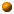Back to Categorical Geometry Homepage
 References BooksAdamek, J. Herrlich, H. Strecker, G. Abstract and Concrete Categories, John Wiley, 1990.Adamek, J. and Rosicky, J. Locally Presentable and Accessible Categories, Cambridge University Press, 1994.Borceux, F. Handbook of Categorical Algebra 1-3. Cambridge University Press, 1994.Diers, Y. Categories of Boolean Sheaves of Simple Algebras, Lecture Notes in Mathematics Vol. 1187, Springer Verlag, Berlin 1986.Diers, Y. Categories of Commutative Algebras, Oxford University Press, 1992.Gabriel, P and Ulmer, F. Lokal prasentierbare Kategorien, Lecture Notes in Mathematics Vol. 221, Springer-Verlarg, Berlin, 1971.Herrlich, H. and Strecker, G. E. Category Theory. Allyn and Bacon, 1973.Johnstone, T. J. Stone Spaces, Cambridge Univ. Press, Cambridge, UK, 1982.MacLane, S. Categories for the Working Mathematician.Springer-Verlag, 1971.MacLane, S. and Moerdijk, I. Sheaves in Geometry and Logic: A First Introduction to Topos Theory. Springer-Verlag,1992. Papers and Books Adamek, J. Herrlich, H. and Strecker, G.  1990.   Abstract and Concrete Categories, John Wiley, New York.  Adamek, J. and Rosicky, J.  1994.   Locally Presentable and Accessible Categories, Cambridge University Press.  Barr, M. and Pare, R.  1980.   Molecular toposes, J. Pure Applied Algebra 17, 127 -152.  Borceux, F.  1994.   Handbook of Categorical Algebra Vol. I - III, Categories and Structures, Cambridge University Press.  Carboni, C. Lack, S. and Walters, R. F. C.  1993.   Introduction to extensive and distributive categories, Journal of Pure and Applied Algebra 84, 145-158.  Diers, Y.  1983.   Une description axiomatique des categories de faisceaux de structures algebriques sur les espaces topologiques booleens, Adv. Math., 47, 258-99.  1986.   Categories of Boolean Sheaves of Simple Algebras, Lecture Notes in Mathematics Vol. 1187, Springer Verlag, Berlin.  1992.   Categories of Commutative Algebras, Oxford University Press.  Eisenbud, D.  1995. Commutative Algebra with a view toward Algebraic Geometry, Springer Verlag, Berlin.  Gabriel, P and Ulmer, F.  1971 Lokal prasentierbare Kategorien, Lecture Notes in Mathematics Vol. 221, Springer-Verlarg, Berlin.  Grothendieck, A. and Dieudonne, J. EGA I Elements de Geometrie Algebrique, I, Grundlehren 166, Springer-Verlag, Heidelberg (new ed., 1971), ix +466 pp. Grothendieck, A. et al. . SGA4 (with Artin, M. and Verdier, J. L.) Theorie des Topos et Cohomologie Etale des Schemas, Lecture Notes in Math. 269, 270, 305, Springer-Verlag, Heidelberg (1972-1973). Hartshorne, R  1977 Algebraic Geometry, Springer-Verlag, Heidelberg.  Hakim, M.  1972 Topos Annelés et Schémas Relatifs. Springer-Verlag, Heidelberg  Johnstone, T. J.  1982 Stone Spaces, Cambridge Univ. Press, Cambridge, UK.  Luo, Z.  1995a. On the geometry of metric sites, Journal of Algebra 176, 210-229.  1995b. On the geometry of framed sites, preprint.  1996 Unitary Categories, Categorical Geometry Homepage, http://www.azd.com. 1997a. Abstract Algebraic Geometry, Categorical Geometry Homepage, http://www.azd.com.  1997b. The language of analytic categories, Categorical Geometry Homepage, http://www.azd.com  1998 Categorical Geometry, on line book, Categorical Geometry Homepage, http://www.azd.com  Mac Lane, S. 1971 Categories for the Working Mathematician, Springer-Verlag, Heidelberg.  Mac Lane, S. and Moerdijk, I.  1993 Sheaves in Geometry and Logic, Springer-Verlag, Heidelberg (1993).  Matsumura, H.  1980, Commutative Algebra,  2nd edition, W. A. Benjamin Co., New York. (Top of Page) Comments Local Categories and Metric Sites: The concept of a metric topology formalizes the passage from spectrums to schemes (i.e. the completion a strict metric site), as well as the theory of fibre products of schemes. After finishing  On the Geometry of Metric Sites, which was later published in J. Algebra in 1995, I found a copy of S. Eilenberg's lecture notes Foundation of Fibre Bundles (University of Chicago), based on the joint work with H. Cartan, in which  the notion of a local category is given. Basically a local category is a category together with a functor to the category of topological spaces such that the pullback sieve of any open sieve is represented by a mono whose image is an open embedding, and the map between objects can be defined locally. Thus a local category is essentially a strict effective metric site. Their theorem 5.1 of  the "2nd enlargement" of  a local category is a special case of the completion of a strict metric site. (Top of Page) Unitary Categories: In the summer of 1996, I wrote two papers on categorical geometry, both based on Diers's book on Zariski categories. One is Unitary Categories, the other one eventually became the first two chapters of Categorical Geometry. The first one is from algebraic point view, and the main notion is an ideal (i.e. congruence). The second one is from geometric point view, and the main notion is a regular mono. Since there is a Galois correspondence between ideals and regular monos (at least when the category is an analytic geometry such that any strong mono is regular), the invariants (such as spectrums in Introduction, or spectrums in Prime Ideals) derived from these two approaches are essentially the same. This is one of the main theorem of categorical geometry.